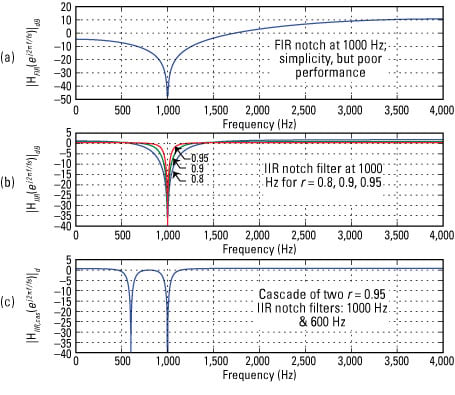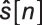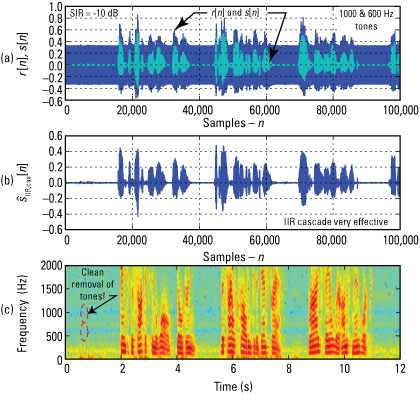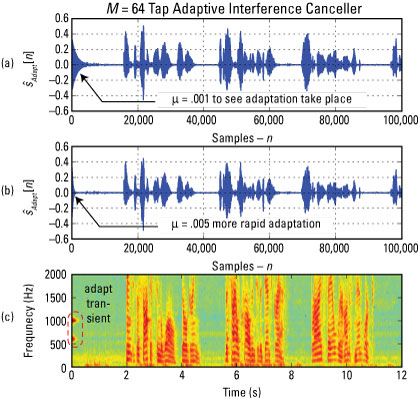##### Signals and Systems For DummiesSimulate Options a, b, and c by using a speech file of a male voice reading phrases. The file enters the simulation as a wav file:`In [<b>685</b>]: fs,s = ssd.from_wav('OSR_us_000_0030_8k.wav')`

You can generate the combination SOI + SNOI by using a custom function that returns r given the speech input s, the signal-to-interference ratio (SIR) in dB — μ = 0.005 10log10(SIR)— the number of samples to process, an array of interference frequencies, and the sampling rate.

`r = soi_snoi_gen(s,SIR_dB,N,fi,fs = 8000)`

In this case, SIR is fixed at –10 dB, meaning that the power in the SNOI is 10 times the power of the SOI! Sinusoids at 1,000 and 600 Hz are screaming in your ears when you’re trying to listen to someone talk to you; how pleasant is that?

The FIR and IIR filters process r at the IPython command line by using the SciPy function signal.lfilter(b,a,r). You can then implement the adaptive filter by using the following custom function:

`n,r,r_hat,e,<u>ao</u>,F,<u>Ao</u> = lms_ic(r,M,<u>mu</u>,delta=1)`

This function returns a large number of ndarrays:

• e, the error signal, e[n] is also• The array ao is the final set of filter coefficients.

• F is a frequency array used to plot the filter frequency response magnitude in dB, found in Ao.

You can get a combination frequency-domain and time-domain view via the spectrogram. The spectrogram plots the short-term spectral estimate of the signal vertically versus time. Bright colors indicate spectral intensity or height above the background.

The figure contains three subplots: s[n] overlaid with r[n] at –10 dB SIR (Figure a),the output of the IIR notch cascade by using r = 0.95 for both sections (Figure b), and spectrogram plots of the IIR notch cascade output (Figure c).Credit: Illustration by Mark Wickert, PhD

The SNOI is powerful when viewed in the time domain (Figure a). Figure b shows that the cascade of IIR notch filters performs a clean excision of the two SNOI tones. The spectrogram (Figure c) confirms what’s happening in the frequency domain. The IIR notch removes just a very narrow swath of frequencies, thereby leaving the SOI largely intact.

You can export the simulation output from Python as wav files by using ssd.to_wav('test_FIR_IIR.wav',fs,s_hat). A listening test is far better than what I can do with graphs in this book, so try some experiments on your own.

The following figure provides both time- and frequency-domain results for the adaptive filter, which is formally known as an adaptive interference canceller (AIC). The plots for (a) and (c) show the remarkable ability of the AIC to quickly converge to filter coefficients that remove the SNOI’s 1,000- and 600-Hz tones.

The parameter μ was reduced to 0.001 to slow down the convergence process and make it visible in the plot of Figure a. The spectrogram plot of Figure c shows the short blip of SNOI tones then convergence and tone excision.Credit: Illustration by Mark Wickert, PhD

Hearing is the final proof. The Python code is in the module ssd.py. The speech file OSR_us_000_0030_8k.wav is also available. Find both online.